cycleRtools is a package intended to open up the power of R to cyclists and those interested in cycling data. Functions are provided for reading raw data files into the R environment–a signficant barrier in any case–as well as several specialist functions that are provided by other, often proprietary platforms.

# Reading in data

For these examples we’ll be using a preloaded dataset, named intervaldata (i.e data(intervaldata)). The corresponding Garmin .fit file is provided in the extdata directory of this package, tarballed together with other files of various formats. See ?read_ride and the example therein.

Briefly, if intervaldata.fit is the file name, then this dataset was generated via:

intervaldata <- read_ride("intervaldata.fit", format = TRUE,
CP = 310,  # Critical power.
sRPE = 7)  # Session RPE.

Note that read_ride() is a generic wrapper for all read_* functions in this package (read_fit(), read_srm() etc…) and calls the appropriate function according to file extension.

## CP and sRPE arguments

When these (numeric) arguments are supplied, they are associated with the parsed data and subsequently used by other functions in this package; they can also serve as useful records should the object be saved as part of ongoing training monitoring. They correspond to “Critical Power” and “session RPE”, respectively. For more information on these metrics see Jones et al., 2010 and Foster et al., 2001.

## The ‘cycleRdata’ format

Calling any read_* function with the argument format = TRUE will generate a data.frame-type object of class cycleRdata. The creation of this class was deemed necessary so that certain columns could be assumed to exist in the data; thus ultimately making life more straightforward for the user. See ?cycleRdata for details of the format.

# Exploring the data

It goes without saying that once these cycling data are within the R environment, endless analytical procedures become available. For the purposes of this demonstration, only some of those procedures facilitated by this package are shown.

## Visualising the data

As is customary, data should first be plotted. cycleRdata objects have an associated plot method:

plot(x = intervaldata,     # "x" is the data, for consistency with other methods.
y = 1:3,              # Which plots should be created? see below.
xvar = "timer.min",   # What should be plotted on the x axis?
xlab = "Time (min)",  # x axis label.
laps = TRUE,          # Should different laps be coloured?
breaks = TRUE)        # Should stoppages in the ride be shown?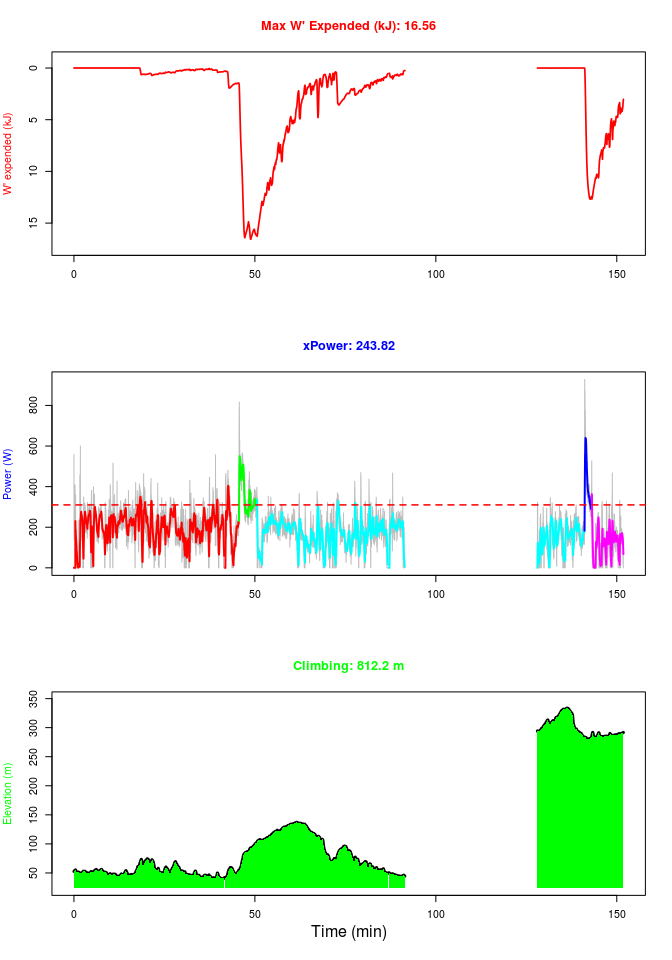The y argument for this plot method specifies the way in which the plot stack should be generated. Specifically length(y) determines the number of plots to be stacked, and the combination of c(1, 2, 3) controls what is plotted and where. As is described in ?plot.cycleRdata, numbers in this argument give:

1. A W’ balance plot (Skiba et al., 2012).
2. A power plot.
3. An elevation plot.

So for example:

plot(intervaldata, y = c(3:1))  # Inverts the above plot.
plot(intervaldata, y = 2)       # Just plots power.
plot(intervaldata, y = c(1, 3)) # W' balance over elevation.

Plots can also be zoomed, and the title metric will be adjusted accordingly.

## Zoom to 0-50 minutes.
plot(intervaldata, y = 3, xvar = "timer.min", xlim = c(0, 50))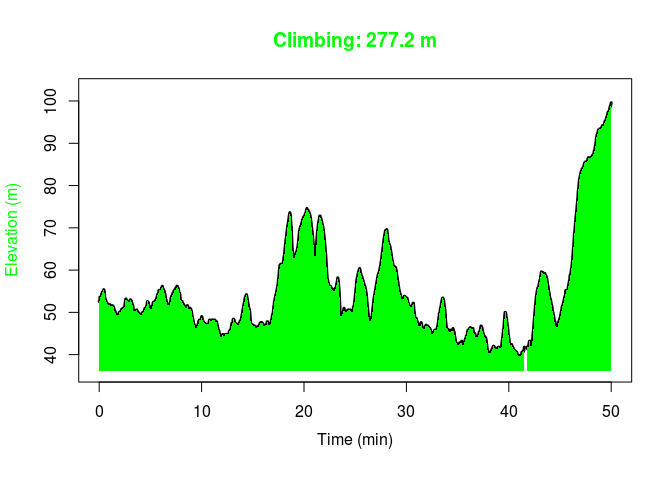## Time in zones

Another want of cyclists is the ability to analyse “time in zones”. This is provided primarily by the functions zone_time() and zdist_plot().

### zone_time()

zone_time(data = intervaldata,
column = power.W,           # What are we interested in?
zbounds = c(100, 200, 300), # Zone boundaries.
pct = FALSE) / 60           # Output in minutes.
## Zone 1 Zone 2 Zone 3 Zone 4
##     22     41     39     14
## How about time above and below CP, as a percentage?
## NB: column = power.W is the default.
zone_time(intervaldata, zbounds = 310, pct = TRUE) 
## Zone 1 Zone 2
##     90     10

### zdist_plot()

zdist_plot(data = intervaldata,
binwidth = 10,               # 10 Watt bins.
zbounds = c(100, 200, 300),  # Zone boundaries.
xlim = c(50, 400))           # Zoom to 50-400 Watts.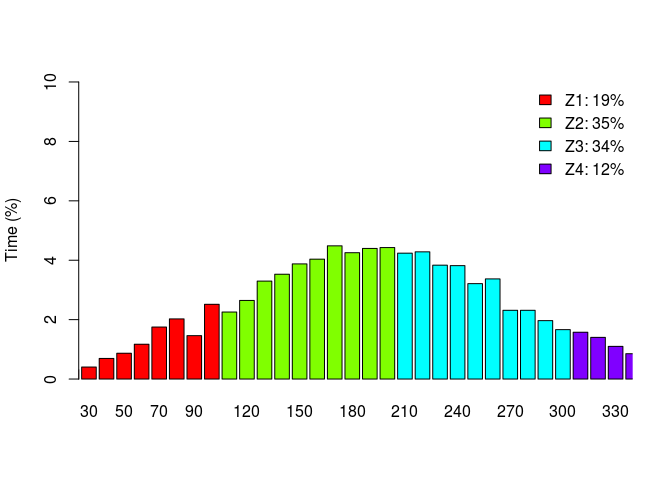## Summarising the data

cycleRdata objects have a summary method, which calculates common metrics and will produce a lap and/or interval summary as appropriate.

summary(intervaldata)
##
## Date:       05 Jan 2016
## Ride start: 09:15:08
##
## **SUMMARY**
##
## ride.time.min     114
## elapsed.time.min  152
## distance.km        52
## work.kJ          1296
## norm.work.kJ     1673
## climbing.m        812
## sRPE.score        801
## TRIMP.score       104
## speed.mean.kmh     27
## speed.sd.kmh        7
## power.mean.W      189
## power.sd.W        108
## xPower.W          244
## power.max.W       928
##
## **POWER-TIME PROFILE**
##
##        Watts
## 1 min    524
## 2 min    430
## 5 min    359
## 10 min   272
## 15 min   256
## 20 min   235
##
## **LAPS**
##
##  # time.min distance.km work.kJ climbing.m speed.mean.kmh speed.sd.kmh
##  1     45.2        20.7     544        228             27          6.2
##  2      5.0         2.5     108         53             30          3.2
##  3     53.5        24.2     533        481             27          7.4
##  4      2.0         1.3      51         10             38          5.6
##  5      8.6         3.3      60         40             23          6.5
##  power.mean.W power.sd.W xPower.W power.max.W
##           200         98      227         600
##           359        106      387         817
##           166         83      194         469
##           425        153      460         928
##           116         99      161         468

Also see ?summary_metrics for other common metrics.

### Power profiling

Best mean powers for specified durations can be generated via the mmv() function. This will return both the best recorded values, as well as when those were recorded in the ride (seconds).

times_sec <- 2:20 * 60   # 2-20 minutes.
prof <- mmv(data = intervaldata,
column = power.W,    # Could also use speed.kmh.
windows = times_sec)
print(prof)
##                  120  180  240  300  360  420  480  540  600  660  720
## Best mean value  430  390  368  359  335  298  291  285  272  263  263
## Recorded @      2734 2736 2735 2735 2677 2645 2537 2511 2437 2363 2316
##                  780  840  900  960 1020 1080 1140 1200
## Best mean value  262  257  256  249  245  243  240  235
## Recorded @      2257 2197 2136 2077 2017 1957 1897 2243

This returns the usual, hyperbolic power-time profile.

hypm <- lm(prof[1, ] ~ {1 / times_sec})  # Hyperbolic model.

## Critical Power (Watts) and W' (Joules) estimates
hypm <- setNames(coef(hypm), c("CP", "W'"))
print(hypm)
##    CP    W'
##   227 28454
## Plot with the inverse model overlaid.
plot(times_sec, prof[1, ], ylim = c(hypm["CP"], max(prof[1, ])),
xlab = "Time (sec)", ylab = "Power (Watts)")
curve((hypm["W'"] / x) + hypm["CP"], add = TRUE, col = "red")
abline(h = hypm["CP"], lty = 2)
legend("topright", legend = c("Model", "CP"), bty = "n",
lty = c(1, 2), col = c("red", "black"))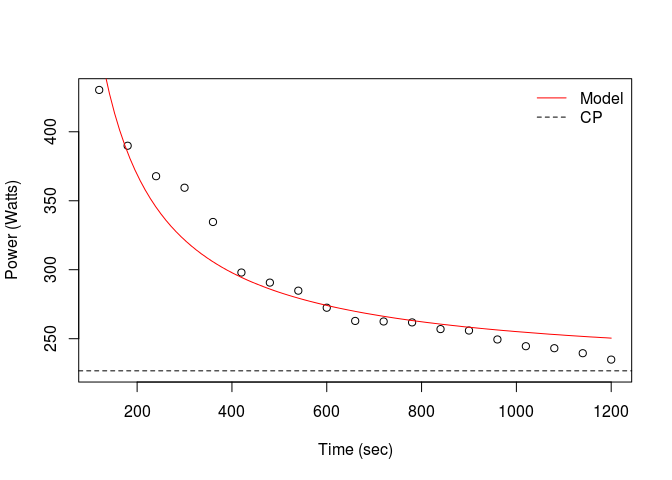### Pt_model()

More sophisticated modelling is provided by the Pt_model function; see ?Pt_model. Briefly:

ms <- Pt_model(prof[1, ], times_sec)
print(ms)
##                                        formula  RSE fit
## Inverse                28453.749 / x + 226.732 16.6
## Exponential      287.697 * e^-0.003x + 232.806  6.4  **
## Power              1613.396 * x^-0.272 - 1.239  8.3
## Three-param 86157.002 / (x + 211.465) + 173.43  7.3
plot(times_sec, prof[1, ], ylim = c(hypm["CP"], max(prof[1, ])),
xlab = "Time (sec)", ylab = "Power (Watts)")
## Showing an exponential model, as it best fits these data.
curve(ms$Pfn$exp(x), add = TRUE, col = "red")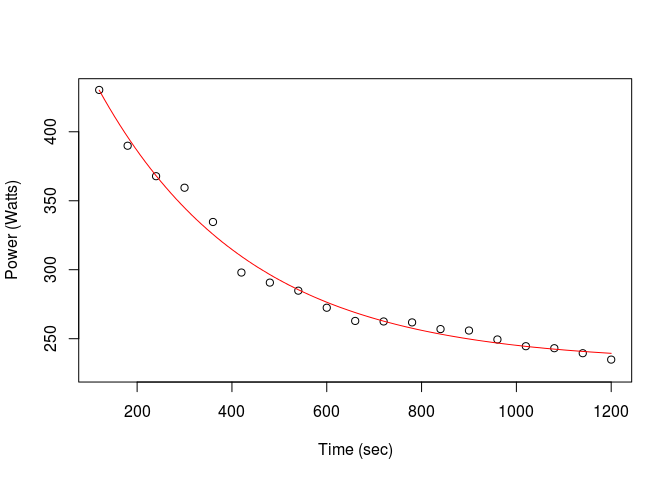## Mapping

Viewing a route map is simple thanks to the leaflet package.

library(leaflet)
leaflet(intervaldata) %>% addTiles() %>% addPolylines(~lon, ~lat)